### Alternate Labeling of the Plane Wave Solutions

Start from the four plane wave solutions: 1 and 2 with positive energy and 3 and 4 with negative. There are four solutions for each choice of momentum.Concentrate on the exponential which determines the wave property. For solutions 3 and 4, both the phase and group velocity are in the opposite direction to the momentum, indicating we have a problem that was not seen in non-relativistic quantum mechanics.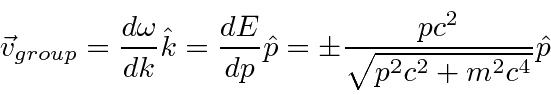Clearly, we want waves that propagate in the right direction. Perhaps the momentum and energy operators we developed in NR quantum mechanics are not the whole story.

For solutions 3 and 4, pick the solution for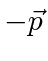to classify with solutions 1 and 2 with momentumwrite everything in terms of the positive square root.We have plane waves of the formwhich is not very surprising. In fact we picked the + sign somewhat randomly in the development of NR quantum mechanics. For relativistic quantum mechanics, both solutions are needed. We have no good reason to associate the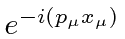solution with negative energy. Lets assume it also has positive energy but happens to have the - sign on the whole exponent.

Consider the Dirac equation with the EM field term included. (While we are dealing with free particle solutions, we can consider that nearly free particles will have a very similar exponential term.)Theoperating on the exponential produces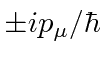. If we change the charge on the electron from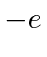toand change the sign of the exponent, the equation remains the same. Thus, we can turn the negative exponent solution (going backward in time) into the conventional positive exponent solution if we change the charge to. Recall that the momentum has already been inverted (and the spin also will be inverted).

The negative exponent electron solutions can be recast as conventional exponent positron solutions.

Jim Branson 2013-04-22